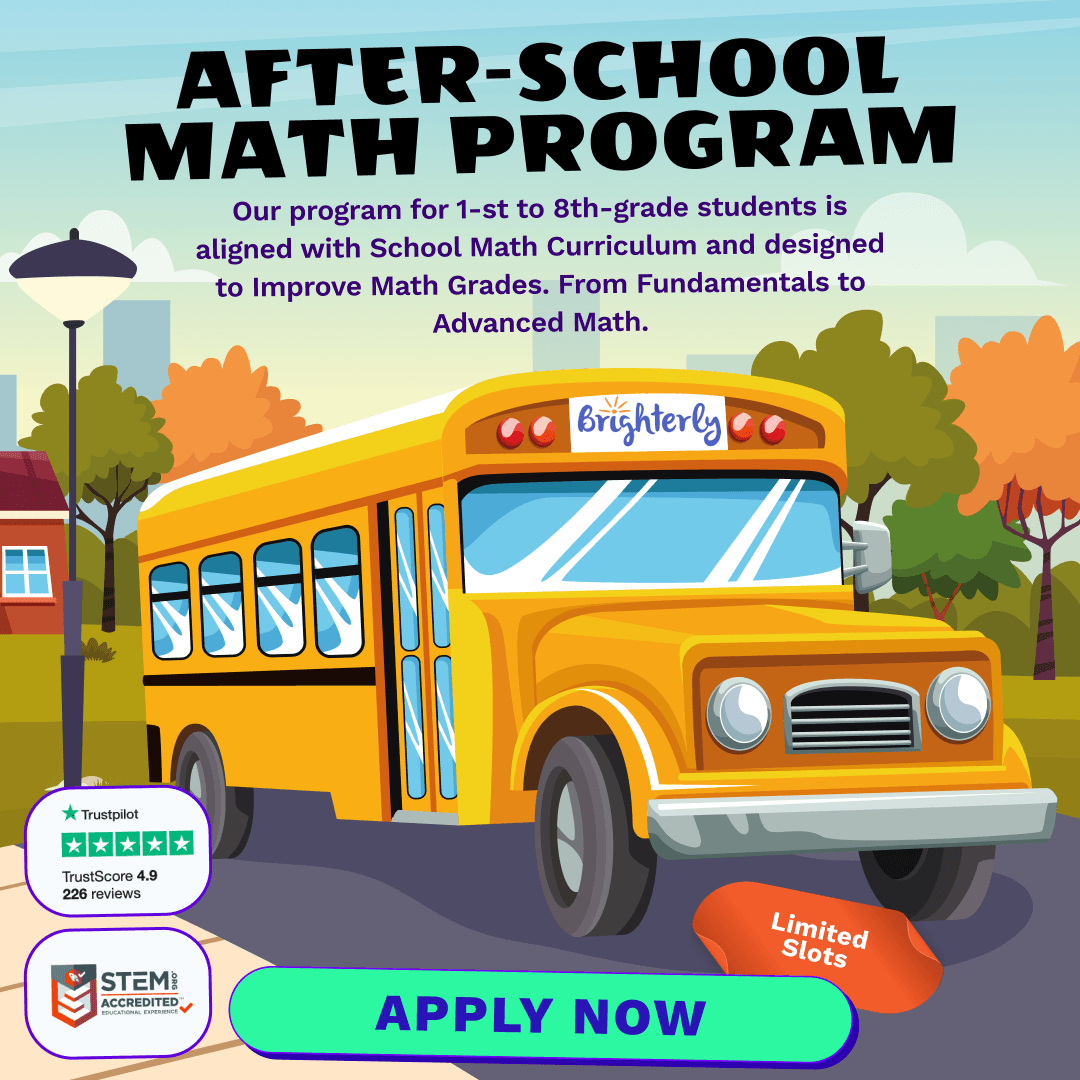# Basic Subtraction Math Practice Test for 2nd Grade – [Medium]

Welcome to Brighterly – where learning math becomes a delightful journey for your young mathematicians. Today, let’s dive deep into the fascinating world of basic subtraction. Tailored specifically for 2nd graders, this guide promises to make subtraction simple, fun, and intuitive.

## Understanding the Basics of Subtraction

At its core, subtraction is the process of taking one number away from another. Imagine you have 10 candies and you give 2 to your friend. How many are left? That’s where subtraction comes into play.

• Start with the bigger number (the total number of candies you had) – this is the minuend.
• Next, identify how many you’re giving away or removing – this is the subtrahend.
• The answer you get after subtracting is called the difference.

## Strategies to Simplify Subtraction

Once the foundation is laid, it’s time to introduce kids to strategies that make subtraction effortless.

1. Counting Backward: Start from the minuend and count backward as many numbers as the subtrahend. For instance, for 10 – 3, start at 10 and count three numbers back to get 7.
2. Using Addition: This might sound counterintuitive, but subtraction is the opposite of addition. If you know that 5 + 3 = 8, then 8 – 5 = 3 becomes easier to understand.
3. Break It Down: For numbers that seem intimidating, break them down. For 15 – 7, think of subtracting 5 first and then 2. Practice worksheets can be a great way to reinforce this strategy.

## Embracing Mistakes and Learning from Them

Every subtraction mistake is a learning opportunity. It’s crucial for kids, especially in 2nd grade, to understand that it’s okay to make errors. The key is to figure out where they went wrong and how to correct it. Brighterly offers interactive math games where kids can practice subtraction and get instant feedback.

## Engage with Fun Subtraction Activities

Turn math into a delightful adventure. Here are some fun ways to practice subtraction:

• Subtraction Bingo: Prepare bingo cards with subtraction problems. The answers get called out, and players mark them.
• Subtraction Treasure Hunt: Place subtraction problems around the house. As kids solve them, they move closer to a hidden treasure.
• Interactive Subtraction Apps: Explore Brighterly’s math apps designed to make subtraction engaging with colorful graphics and interactive challenges.

To sum it up, subtraction is more than just numbers. It’s about understanding concepts, applying strategies, and learning from mistakes. With Brighterly, subtraction becomes a joyous journey, full of discoveries and achievements. So, let’s subtract the fear and add some fun to math!

Basic Subtraction Practice Test for 2nd Grade

Get ready for math lessons with Brighterly! Dive into a collection of carefully curated subtraction problems designed to challenge and hone your child's mathematical skills.

1 / 20

What is 15 - 7?

2 / 20

Subtract the smallest from the biggest: 10, 7, 5, 2

3 / 20

What do you get when you subtract 18 from 25?

4 / 20

If you had 20 candies and gave 11 away, how many would you have left?

5 / 20

Tom has 13 apples. He ate 5. How many does he have now?

6 / 20

There were 17 balloons. 8 flew away. How many remained?

7 / 20

Lily had 24 stickers and gave 9 to her friend. How many stickers does she have now?

8 / 20

There are 12 ducks in the pond. If 4 swam away, how many ducks are left?

9 / 20

Jenny read 19 pages of her book and has 9 pages left. How many pages does the book have in total?

10 / 20

16 - 8 = ?

11 / 20

Mike found 22 seashells and lost 7. How many does he have now?

12 / 20

Subtract 11 from 23.

13 / 20

A box had 14 crayons. 6 were red and the rest were blue. How many blue crayons are there?

14 / 20

If a train has 20 compartments and 7 are for cargo, how many are for passengers?

15 / 20

21 - 13 = ?

16 / 20

Liam baked 18 cookies. He ate 6 and gave 5 to his friend. How many cookies are left?

17 / 20

What is 15 - 5?

18 / 20

There were 26 birds on the tree. 12 flew away. How many birds remained?

19 / 20

What is the result of 17 minus 9?

20 / 20

Tina bought 23 candies. She ate 10 and gave 2 to her brother. How many candies does Tina have left?

0%
Poor Level
Weak math proficiency can lead to academic struggles, limited college, and career options, and diminished self-confidence.
Mediocre Level
Weak math proficiency can lead to academic struggles, limited college, and career options, and diminished self-confidence.
Needs Improvement
Start practicing math regularly to avoid your child`s math scores dropping to C or even D.
High Potential
It's important to continue building math proficiency to make sure your child outperforms peers at school.

After-School Math Programs• Our program for 1st to 8th grade students is aligned with School Math Curriculum.

After-School Math Programs
Our program for 1st to 8th grade students is aligned with School Math Curriculum.